# OliNo

Renewable Energy

## CDE Technology BV Ledion Lighting A55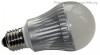presents a led light bulb in de form of a normal incandescent light bulb. It is a very robust light bulb. This article shows the measurement results. Many parameters are also found in the Eulumdat file.

See this overview for a comparison with other light bulbs.

### Summary measurement data

parameter meas. result remark
Color temperature 5904 K Cold white.
Luminous intensity Iv 51 Cd
Beam angle 138 deg
Power P 5.4 W
Power Factor 0.55 For every 1 kWh net power consumed, there has been 1.5 kVAhr for reactive power.
Luminous flux 229 lm
Luminous efficacy 43 lm/W
CRI_Ra 74 Color Rendering Index.
Coordinates chromaticity diagram x=0.3240 and y=0.3343
Fitting E27
PAR-value 0.47 μMol/s/m2 The number of photons seen by an average plant when it is lit by the light of this light bulb. Valid at 1 m distance of the light bulb.
D x H external dimensions 60 x 115 mm External dimensions of the light bulb.
D x L luminous area 60 x 30 mm Dimensions of the luminous area (used in an Eulumdat file). It is the matt glass part’s diameter and height.
General remarks The ambient temperature during the whole set of measurements was 21.5 deg C.
Warm up effect: during the warm up time the illuminance decreases with 8 %.

Voltage dependency: ttbche power consumption and illumination are not significantly dependent from the used voltage.

Measurement report (PDF)Eulumdat fileRight click on icon and save the file.

### Eulumdat light diagram

An interesting graph is the light diagram, indicating the intensity in the C0-C180 and the C90-C270 plane. This light diagram below comes from the program Qlumedit, that extracts these diagrams from an Eulumdat file.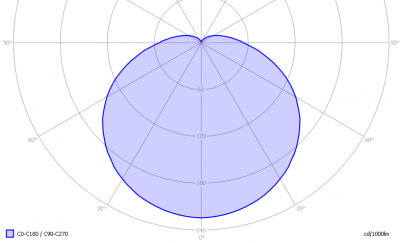The light diagram giving the radiation pattern.

It indicates the luminous intensity around the light bulb. Th C0-C180 plane and C90-C270 plane are the same, as the light bulb is symmetrical over its z-axis (height axis).

The light bulb emits the light all around (albeit a little bit towards the back side).

The unit is Cd/1000lm, meaning the intensity in Cd assuming there would be 1000 lumen in the measured light bulb. This enables comparing different types of light bulbs.

### Illuminance Ev at 1 m distance, or luminous intensity Iv

Herewith the plot of the averaged luminous intensity Iv as a function of the inclination angle with the light bulb.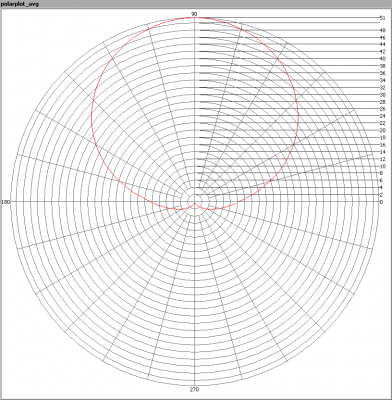The radiation pattern of the light bulb.

This radiation pattern is the average of the light output of the light diagram given earlier. Also, in this graph the luminous intensity is given in Cd.

These averaged values are used (later) to compute the lumen output.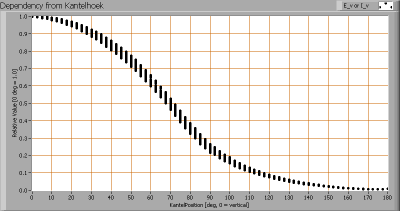Intensity data of every measured turn angle at each inclination angle.

This plot shows per inclination angle the intensity measurement results for each turn angle at that inclination angle. There are little differences in illuminance values for different turn angles. However for further calculations the averaged values will be used.

When using the average values per inclination angle, the beam angle can be computed, being 138 degrees. This is the average value which is not valid for all C-planes. In the light diagram at the top, it can be seen that this average value lies close to the value of the C90-C270 plane.

### Luminous flux

With the averaged illuminance data at 1 m distance, taken from the graph showing the averaged radiation pattern, it is possible to compute the luminous flux.

The result of this computation for this light spot is a luminous flux of 229 lm.

### Luminous efficacy

The luminous flux being 229 lm, and the power of the light bulb being 5.4 W, yields a luminous efficacy of 43 lm/W.

A power factor of 0.55 means that for every 1 kWh net power consumed, a reactive component of 1.5 kVAr was needed.

 Light bulb voltage 230 VAC Light bulb current 42 mA Power P 5.4 W Apparent power S 9.8 VA Power factor 0.55

Of this light bulb the voltage across ad the resulting current through it are measured and graphed.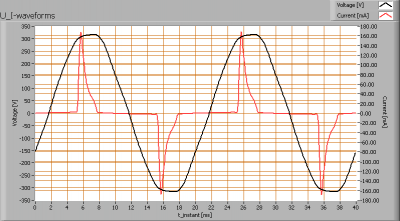Voltage across and current through the lightbulb

There are known peaks in the current, just before the voltage gets to it maximum value. This is a well known power supply design, making use of a capacitor to store the energy while the voltage is high and release it when the voltage drops.

The current form is not symmetrical nor has a sine form, hence the power factor is low.
Also the power spectrum of the current is determined.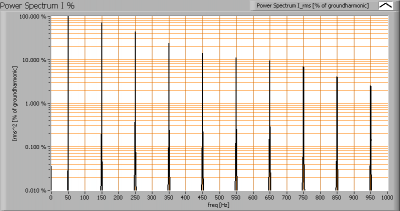Current power spectrum in % of the first harmonic (50 Hz).

The abrupt current peaks resut in a high amount of higher harmonics.

### Color temperature and Spectral power distribution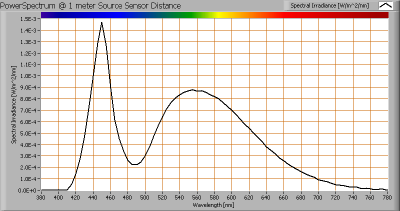The spectral power distribution of this light bulb. Spectral values valid at 1 m distance from the light bulb.

The measured color temperature is about 5900 K which is cold white.

This color temperature is measured straight underneath the light bulb. Below a graph showing the color temperature for different inclination angles.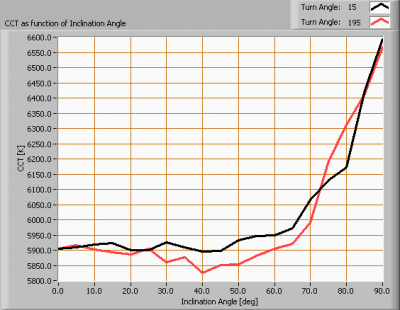Color temperature as a function of inclination angle.

The color temperature is given for inclination angles up to 90 degrees; more ha not been measured.

Up to 70 degrees inclination angle the color temperature stays within 2 %. Then it increases.

### PAR value and PAR spectrum

To make a statement how well the light of this light bulb is for growing plants, the PAR-area needs to be determined. PAR stands for Photosynthetic Active Radiation which is that radiation that participates actively to photosynthesis and is expressed in μMol/s/m2.

Photosynthesis is the essence for growth and flowering of plants, where the blue part of the light spectrum is responsible for growth and the red part is responsible for flowering and budding (for gemmation). For photosynthesis it is more important to count the number of photons rather than the power in the light.
The power spectrum (power per wave length) of the light of the light bulb is converted into the number of photons (number of photons per wave length) and then these number of photons is weighted against the relative sensitivity of an average plant (which varies in value dependent on the wave length). The relative sensitivity curve in DIN-norm 5031-10:2000 is used for this. The next image shows the result.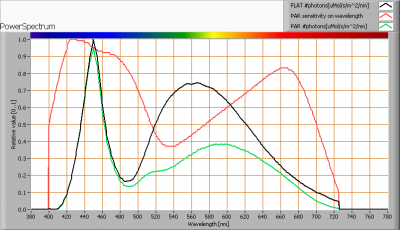The photon spectrum, then the sensitivity curve and as result the final PAR spectrum of the light of this light bulb

The black curve gives the power spectrum of the light of this light bulb, in number of photons per wave length.

In red the relative sensitivity curve of an average plant (according to DIN norm 5031-10:2000).

Multiplying these two results in a curve showing the number of photos per wave length of the light of this light bulb, the PAR spectrum.

Summing all the photons, gives a PAR number that for this light results in 0.47 μMol/s/m2. This value is valid at 1 m distance of the light bulb.

Dividing the PAR number by the total number of photons of the light of this light bulb gives a sort of PAR efficiency, which is 65 % (valid for the PAR wave length range of 400 – 725 nm). So 65 % of the photons is effectively used by the average plant.

Note: when mentioning this percentage of efficiency, it is important to verify if all wavelengths are present in a sufficient manner. Not that only blue light is available, when the plant is going to be used for flowering where specifically red light is needed.

### Chromaticity diagram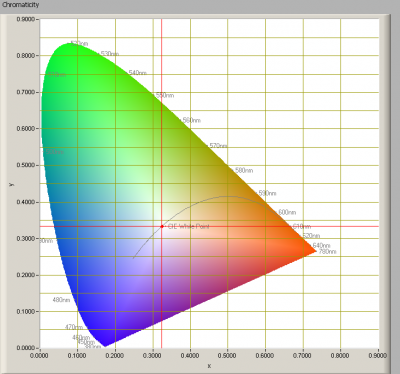The chromaticity space and the position of the lamp’s color coordinates in it.

The light coming from this lamp is a little below the Planckian Locus (the black path in the graph).

Its coordinates are x=0.3240 and y=0.3343.

### Color Rendering Index (CRI) or also Ra

Herewith the image showing the CRI as well as how well different colors are represented (rendered). The higher the number, the better the resemblance with the color when a black body radiator would have been used (the sun, or an incandescent lamp).

Each color has an index Rx, and the first 8 indexes (R1 .. R8) are averaged to compute the Ra which is equivalent to the CRI.CRI of the light of this lightbulb.

The value of 74 is lower than 80 which is considered a minimum value for indoor usage.

Note: the chromaticity difference is 0.0027 indicates the distance to the Planckian Locus. Its value is lower than 0.0054, which means that the calculated CRI result is meaningful.

### Voltage dependency

The dependency of a number of lamp parameters on the lamp voltage is determined. For this, the lamp voltage has been varied and its effect on the following lamp parameters measured: illuminance E_v [lx], the lamppower P [W], the (Correlated) Color Temperature [K] and the luminous efficacy [lm/W].Lamp voltage dependencies of certain light bulb parameters, where the value at 230 V is taken as 100 %.

There is no significant variation of parameters when the voltage varies in the 200 – 250 V range.

When the voltage at 230 V varies with + and – 5 V abruptly, then the illuminance varies within 0.1 %, so when abrupt voltage changes occur this effect is not visible in the illuminance output.

### Warm up effects

After switch on of a cold lamp, the effect of heating up of the lamp is measured on illuminance E_v [lx], color temperature CT or correlated color temperature CCT [K], the lamppower P [W] and the luminous efficacy [lm/W].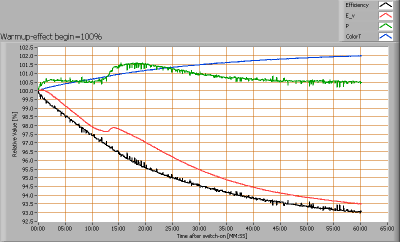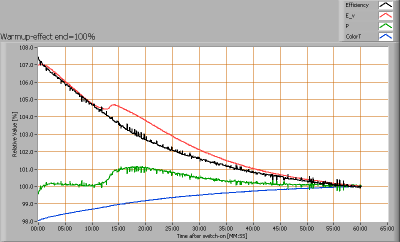Effect of warming up on different light bulb parameters. At top the 100 % level is put at begin, and at bottom at the end.

The warm-up time takes a bit more than one hour and in that time the illumination level decreases with about 8 %.